# SUMIF function in Excel: formula examples for numbers, dates, text, blanks and not blanks

The tutorial shows how to do SUMIF in Excel to conditionally sum cells. Our main focus will be on real-life formula examples with all kinds of criteria including dates, text, numbers, wildcards, blanks and non-blanks.

Microsoft Excel has a handful of functions to summarize large data sets for reports and analyses. One of the most useful functions that can help you make sense of an incomprehensible set of diverse data is SUMIF. Instead of adding up all numbers in a range, it lets you sum only those values that meet your criteria.

## Excel SUMIF function

The SUM function in Excel returns the sum of the values in a range that meet the condition you specify.

It has the following syntax and arguments:

SUMIF(range, criteria, [sum_range])

Where:

• Range (required) - the range of cells to be evaluated by criteria.
• Criteria (required) - the condition that determines which cells will be added. It can be supplied in the form a number, text, date, cell reference, logical expression, or function.
• Sum_range (optional) - the range in which to add up numbers. If omitted, then range is summed.

The function is available in all versions of Excel 365, Excel 2019, Excel 2016, Excel 2013, Excel 2010, and earlier.

## Basic SUMIF formula

For starters, let's build an Excel SUMIF formula in its simplest form.

In the sample table below, supposing you want to get a total of sales for a specific region, say North. To have it done, we define the following arguments:

• Range - a list of regions (B2:B10).
• Criterion - "North" or a cell containing the region of interest (F1).
• Sum_range - the sales amounts to be added up (C2:C10).

Putting the arguments together, we get the following formulas:

`=SUMIF(B2:B10, "north", C2:C10)`

or

`=SUMIF(B2:B10, F1, C2:C10)`

Both only sum the cells for the North region: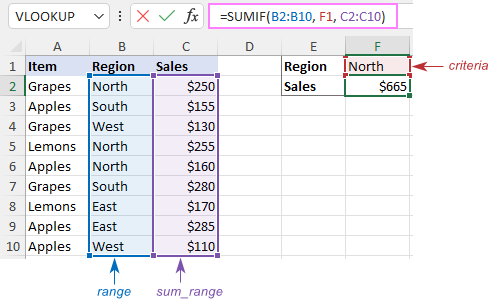## How to use SUMIF in Excel

Looking simple at first sight, SUMIF is quite a tricky function. The following notes will help you better understand its internal logic and avoid common mistakes.

### 1. SUMIF supports only one criterion

The syntax of the SUMIF function has room for only one condition. To sum with multiple criteria, either use the SUMIFS function (adds up cells that meet all the conditions) or build a SUMIF formula with multiple OR criteria (sums cells that meet any of the conditions).

### 2. SUMIF criteria syntax

For criteria, the SUMIF function allows using different data types including text, numbers, dates, cell references, logical operators (>, <, =, <>), wildcard characters (?, *, ~) and other functions. The syntax of such criteria is quite specific.

If the criteria argument includes a text value, wildcard character or logical operator followed by text, number or date, enclose the whole criteria in quotation marks. For example:

`=SUMIF(B2:B10, "north*", C2:D10)`

`=SUMIF(C2:D10, ">100")`

`=SUMIF(B2:B10, "<>north", C2:D10)`

`=SUMIF(C2:C10, "<=9/10/2020", B2:B10)`

When a logical operator is followed by a cell reference or another function, the criteria should be provided in the form of a string. For this, you use an ampersand (&) to concatenate a logical operator and a reference or function. For example:

`=SUMIF(B2:B10, ">"&F1)`

`=SUMIF(C2:D10, "<="&TODAY(), B2:B10)`

Please note that comparison operators are enclosed in quotation marks while cell references are not.

### 3. Range and sum_range should have the same dimensions

For a SUMIF formula to work correctly, the sum_range argument must be the same size as range, otherwise you may get misleading results. The point is that sum_range defines only the upper left cell of the range that will be summed, the remaining area is determined by the size and shape of the range argument.

Given the above, the below formula will actually sum cells in C2:C10 and not in C2:D10. Why? Because range consists of 1 column and 9 rows, and so does sum_range.

`=SUMIF(B2:B10, "north", C2:D10)`

### 4. Range and sum_range cannot be arrays

Though SUMIF can process an array constant in criteria like shown in this example, it does not support arrays in range and sum_range. These two arguments can only be cell ranges.

### 5. SUMIF does not recognize text case

By design, SUMIF in Excel is not case-sensitive, meaning it treats uppercase and lowercase letters as the same characters. To make a case-sensitive SUMIF formula, use the SUMPRODUCT function together with EXACT.

## Excel SUMIF formula examples

Now that you know the main rules of using the SUMIF function in Excel, it's time to apply your knowledge to something practical and improve the skills you've learned.

### Example 1. SUM IF greater than or less than

To sum numbers greater than or less than a particular value, configure the SUMIF criteria with one of the following logical operators:

• Greater than (>)
• Greater than or equal to (>=)
• Less than (<)
• Less than or equal to (<=)

In the range B2:B10, supposing you wish to sum numbers greater than 200. To express this condition, put a comparison operator (>) before the number and surround the construction in double quotes:

`=SUMIF(B2:B10, ">200")`

If the target number is in another cell, say E1, concatenate the logical operator and cell reference:

`=SUMIF(B2:B10, ">"&E1)`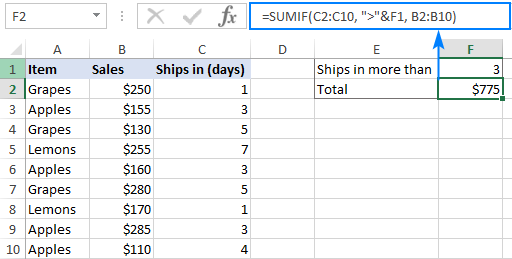In a similar manner, you can sum values smaller than 200 by using the less than (<) operator:

`=SUMIF(B2:B10, "<200")`

### Example 2. SUM IF equal to

A SUMIF formula with the "equal to" criteria works for both numbers and text. In such criteria, the equals sign is not actually required.

For instance, to find a total of the items that ship in 3 days, any of the below formulas will do:

`=SUMIF(C2:C10, 3, B2:B10)`

or

`=SUMIF(C2:C10, "=3", B2:B10)`

To sum if equal to cell, simply supply a cell reference for criteria:

`=SUMIF(C2:C10, F1, B2:B10)`

Where B2:B10 are the amounts, C2:C10 is the shipment duration, and F1 is the desired delivering time.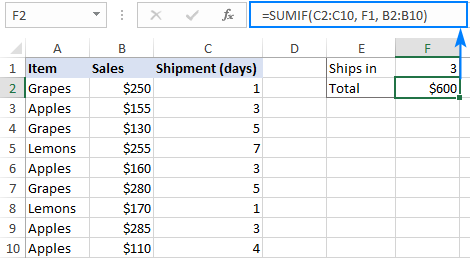Likewise, you can use the "equal to" criteria with text values. For instance, to add up all the Apples amounts, choose any of the below formulas:

`=SUMIF(A2:A10, "apples", B2:B10)`

`=SUMIF(A2:A10, "=apples", B2:B10)`

`=SUMIF(A2:A10, F1, B2:B10)`

Where A2:A10 is the list of items to compare against the value in F1.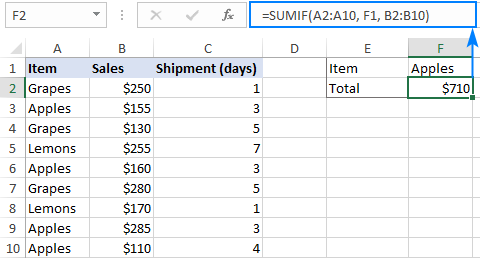Note. The above formulas imply that the criterion matches the entire cell contents. Consequently, the SUMIF function will add up Apples sales but not, say, Green Apples. To sum partial matches, construct the "if cell contains" criteria like in this SUMIF wildcard formula.

### Example 3. SUM IF not equal to

To build the "not equal to" criteria, use the "<>" logical operator.

When a value, either text or number, is hardcoded in the criteria, remember to surround the entire construction by double quotes.

For example, to sum the amounts with shipment other than 3 days, the formula goes as follows:

`=SUMIF(C2:C10, "<>3", B2:B10)`

To find a total of all the items except Apples, the formula is:

`=SUMIF(A2:A10, "<>apples", B2:B10)`

When the criteria value is in another cell, concatenate the "not equal to" operator and a cell reference like this:

`=SUMIF(A2:A10, "<>"&F1, B2:B10)`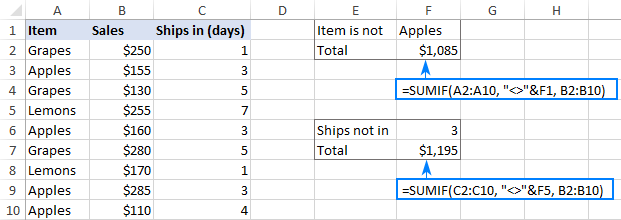### Example 4. SUM IF not blank

To make "if cell is not blank then sum" kind of formula, use "<>" as the criteria. This will add up all cells that contain anything in them, including zero-length strings.

For instance, here's how you can sum sales for all the regions, i.e. where column B is not blank:

`=SUMIF(B2:B10, "<>", C2:D10)`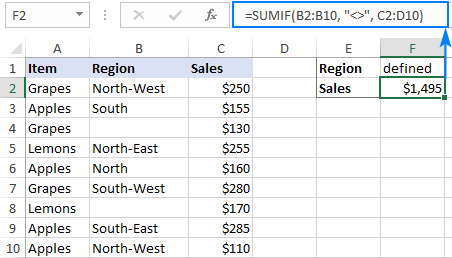### Example 5. SUM IF blank

To sum cells in some column if a corresponding cell in another column is blank, use one of these criteria:

• "" - to sum cells if another cell is blank; cells that contain empty strings are also considered blank.
• "=" - to sum cells that are absolutely empty.

In our sample table, the following formula will get a total of sales for undefined regions, i.e. where a cell in column B is blank:

`=SUMIF(B2:B10, "", C2:D10)`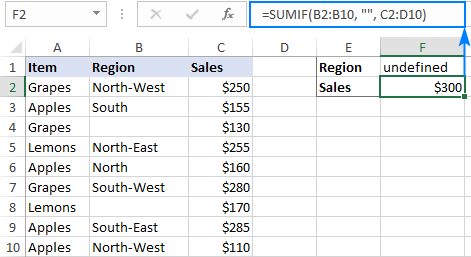### Example 6. Excel SUMIF wildcard

To sum cells based on partial matches, include one of the following wildcard characters in your criteria:

• Question mark (?) to replace any single character.
• Asterisk (*) to substitute any number of characters.

For instance, the below formula will only sum sales for the North region:

`=SUMIF(B2:B10, "north", C2:D10)`

To total sales for all northern regions, including North, North-East, and North-West, put an asterisk right after the text:

`=SUMIF(B2:B10, "north*", C2:D10)`

If you'd rather input the region of interest in a predefined cell (F1), then concatenate a cell reference and a wildcard character enclosed in quotes:

`=SUMIF(B2:B10, F1&"*", C2:D10)`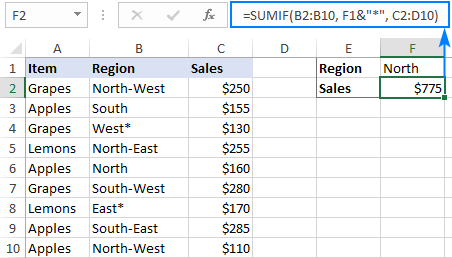To match a literal question mark or asterisk, place a tilde (~) before the character, e.g. "~?" or "~*".

For example, to sum sales for the regions marked with *, use "*~*" for criteria. In this case, the first asterisk is a wildcard and the second one is a literal asterisk character:

`=SUMIF(B2:B10, "*~*", C2:D10)`

If the criteria (* in this case) is supposed to be entered in a separate cell, then concatenate a tilde and the cell reference, like this:

`=SUMIF(B2:B10, "*"&"~"&F1, C2:D10)`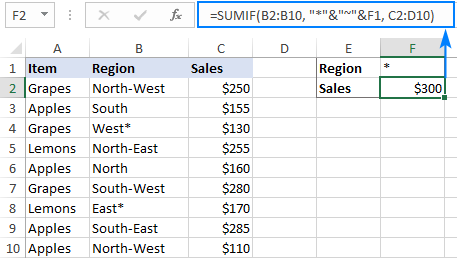### Example 7. Excel SUMIF with dates

Using dates as SUMIF criteria is very much like using numbers. The most important thing is to supply a date in the format that Excel understands. If you are not sure which date format is supported and which is not, the DATE function can be a solution.

Assuming you are looking to sum sales for the items delivered before 10-Sep-2020, the criteria can be expressed in this way:

`=SUMIF(C2:C10, "<9/10/2020", B2:B10)`

or

`=SUMIF(C2:C10, "<"&DATE(2020,9,10), B2:B10)`

or

`=SUMIF(C2:C10, "<"&F1, B2:B10)`

Where F1 is the target date.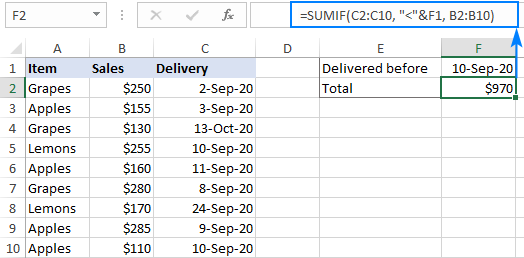To sum cells based on today's date, include the TODAY function in your criteria. For example, the below formula will calculate a total of sales with a delivery date prior to today:

`=SUMIF(C2:C10, "<"&TODAY(), B2:B10)`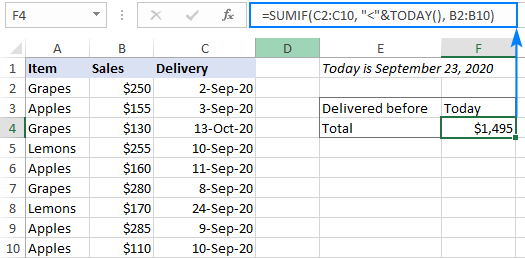To sum within a date range, you need to define a smaller and larger date separately. This can be done with the help of the SUMIFS function that supports multiple criteria.

For example, to sum if a date is between two dates, this is the formula to use:

`=SUMIFS(B2:B10, C2:C10, ">="&F1, C2:C10, "<="&G1)`

Where B2:B10 is the sum range, C2:C10 is the list of dates to check, F1 is the start date and G1 is the end date.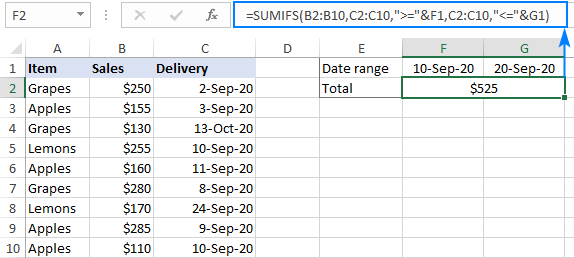More formula examples can be found in SUMIFS with date range as criteria.

## How to do SUMIF from another sheet

When you need to conditionally sum data from a different sheet, simply provide external references for the SUMIF arguments. The easiest way is to start typing a formula, switch to another worksheet and select ranges using the mouse. Excel will insert all the references automatically, without you having to worry about the correct syntax.

For instance, the below formula will add up values in C2:C10 on the Data sheet based on the criteria in B3 on Sheet1:

`=SUMIF(Data!B2:B10, B3, Data!C2:C10)`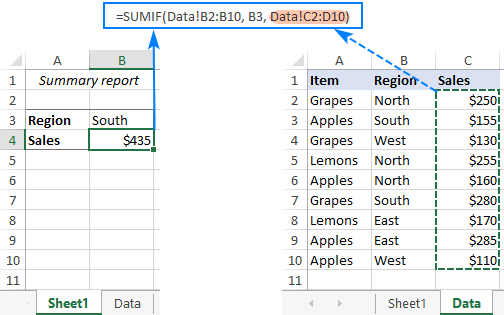## Case-sensitive SUMIF formula in Excel

As already mentioned, the Excel SUMIF function is case-insensitive by nature. To conditionally sum cells considering the letter case, you will have to use a couple of other functions, namely SUMPRODUCT and EXACT that treats uppercase and lowercase as different characters:

SUMPRODUCT(--(EXACT(range, criteria)), sum_range)

Supposing you have a list of item codes in column A, where uppercase and lowercase letters define different items. Your goal is to sum numbers in column B for a specific item, say A-01.

To have it done, you can enter the target item directly in the 2nd argument of the EXACT function:

`=SUMPRODUCT(--(EXACT(A2:A10, "A-01")), B2:B10)`

Or input the item code in a separate cell (E1) as shown in the screenshot:

`=SUMPRODUCT(--(EXACT(A2:A10, E1)), B2:B10)`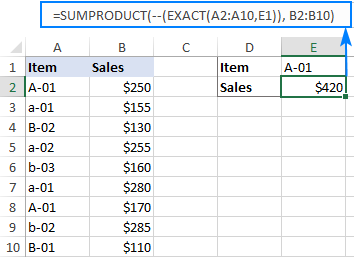How this formula works:

At the heart of the formula, the EXACT function compares the target item against each item in the source list and returns TRUE if an exact match is found, FALSE otherwise:

`{TRUE;FALSE;FALSE;FALSE;FALSE;FALSE;TRUE;FALSE;FALSE}`

A double unary operator (--) converts TRUE and FALSE into 1 and 0, respectively:

`{1;0;0;0;0;0;1;0;0}`

The SUMPRODUCT function multiplies the elements of the above array by the corresponding items in B2:B10:

`SUMPRODUCT({1;0;0;0;0;0;1;0;0}, {250;155;130;255;160;280;170;285;110})`

And because multiplying by 0 gives zero, only the items for which EXACT returned TRUE (1) survive:

`SUMPRODUCT({250;0;0;0;0;0;170;0;0})`

Finally, SUMPRODUCT adds up the products and outputs the sum.

That's how to use SUMIF in Excel. Hopefully, our formula examples have given you some good insights. Anyway, I thank you for reading and hope to see you on our blog next week!

1.Ismail Abdus-Salam says:
•Svetlana Cheusheva (Ablebits.com Team) says: